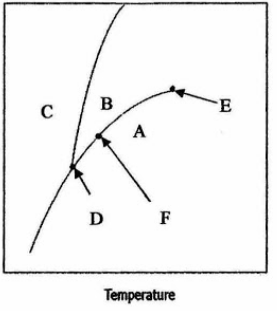# Problem: In the phase diagram of a substance shown  to the right, fill in the blanks to make correct statements. a) Moving from point B to C results in a transition from _______ phase to ________ phase. b) At point _______ all three phases of solid, liquid, and gas coexist in equilibrium. c) At a higher pressure and temperature than point ______ the substance becomes a supercritical fluid. d) At point F, ______ phase and ______ phase coexist in equilibrium.

###### FREE Expert Solution
82% (327 ratings)
###### Problem Details

In the phase diagram of a substance shown  to the right, fill in the blanks to make correct statements.

a) Moving from point B to C results in a transition from _______ phase to ________ phase.

b) At point _______ all three phases of solid, liquid, and gas coexist in equilibrium.

c) At a higher pressure and temperature than point ______ the substance becomes a supercritical fluid.

d) At point F, ______ phase and ______ phase coexist in equilibrium.What scientific concept do you need to know in order to solve this problem?

Our tutors have indicated that to solve this problem you will need to apply the Phase Diagram concept. You can view video lessons to learn Phase Diagram. Or if you need more Phase Diagram practice, you can also practice Phase Diagram practice problems.

What is the difficulty of this problem?

Our tutors rated the difficulty ofIn the phase diagram of a substance shown  to the right, fil...as medium difficulty.

How long does this problem take to solve?

Our expert Chemistry tutor, Sabrina took 2 minutes and 13 seconds to solve this problem. You can follow their steps in the video explanation above.

What professor is this problem relevant for?

Based on our data, we think this problem is relevant for Professor Der Sluys' class at PSU.